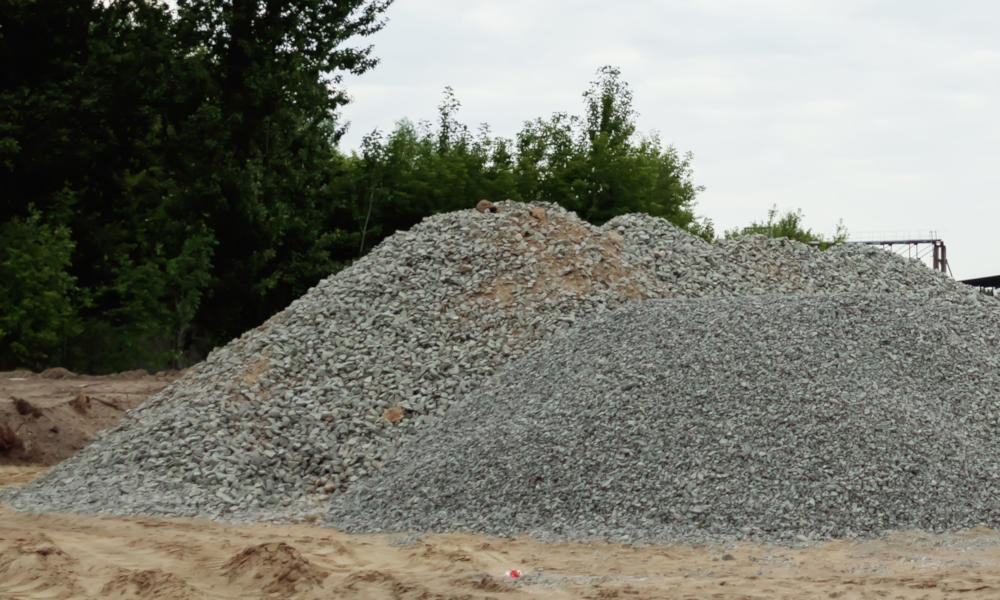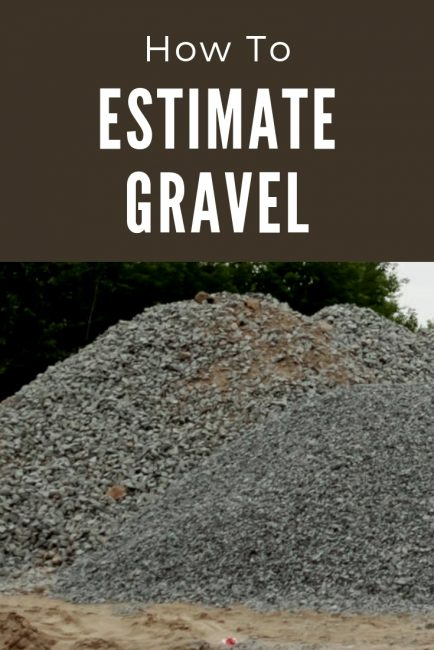# Gravel Calculator – Estimate Landscaping Material in Yards and Tons

Calculate the amount of gravel or aggregate needed in tons and cubic yards by entering the dimensions below. Estimate material given the length/width/depth, the area, or the volume of material needed.

## Calculate Material using Project Dimensions

Optionally enter the price per ton or cubic yard
\$

## Calculate Material using Area and Depth

Find area using our square footage calculator
Optionally enter the price per ton or cubic yard
\$

## Calculate Material using Volume

Find volume using our cubic yards calculator
Optionally enter the price per ton or cubic yard
\$

## Result:

Tons

Cubic Yards
Cost

Total cost
Learn how we calculated this below

## How to Estimate How Much Gravel You Need

Gravel and other landscaping material are often sold either by the ton or the cubic yard, but most suppliers sell large volumes by weight in tons.

To find the weight of material in tons, you’ll need to know the approximate density of the material. Fortunately, there are some common densities that you can use for these calculations. See common weights and densities of landscape material.### Step One: Calculate Volume in Yards

The first step to estimating gravel, sand, or soil is to estimate the volume of material needed. Start by measuring the length, width, and depth of the area you need to fill in the same unit of measurement.

Multiply the length, width, and height together to find the volume of the space. Now, convert the volume to cubic yards.

If the initial measurements were in inches, then convert cubic inches to cubic yards by dividing by 46,656. If the initial measurements were in feet, then divide by 27 to get cubic yards.

As a shortcut, try our cubic yardage calculator to conveniently calculate your volume.

### Step Two: Calculate Gravel Weight in Tons

Armed with the volume of material needed in cubic yards, the weight of material in tons can be found by multiplying the volume by the material density. Most gravel weighs 1.4 to 1.7 tons per cubic yard. See below for more common material densities.

For example, let’s find the amount of gravel needed for a space that is 10 feet long, 10 feet wide, and 1 foot deep.

volume = length × width × depth
volume = 10′ × 10′ × 1′ = 100 cu ft
cu yds = 100 cu ft / 27 = 3.7

weight = cu yds × density
weight min = 3.7 × 1.4 = 5.2 tons
weight max = 3.7 × 1.7 = 6.3 tons

## Weight of Gravel and Other Landscaping Materials

Refer to the chart below for the weights of common landscaping material, including gravel, rock, sand, and soil.

Weight per cubic yard and cubic meter of gravel and other common landscape material
Material Weight per Cubic Yard Weight per Cubic Meter
Pounds Tons Kilograms Metric Tons
Gravel (¼” – 2″) 2,800 – 3,400 lbs 1.4 – 1.7 tons 1,660 – 2,020 kg 1.66 – 2.02 tonnes
Rock (2″ – 6″) 3,000 – 3,400 lbs 1.5 – 1.7 tons 1,780 – 2,020 kg 1.78 – 2.02 tonnes
Sand (wet) 3,000 – 3,400 lbs 1.5 – 1.7 tons 1,780 – 2,020 kg 1.78 – 2.02 tonnes
Sand (dry) 2,600 – 3,000 lbs 1.3 – 1.5 tons 1,540 – 1,780 kg 1.54 – 1.78 tonnes
Topsoil (wet) 3,000 – 3,400 lbs 1.5 – 1.7 tons 1,780 – 2,020 kg 1.78 – 2.02 tonnes
Topsoil (dry) 2,000 – 2,600 lbs 1 – 1.3 tons 1,190 – 1,540 kg 1.19 – 1.54 tonnes
Riprap 3,400 – 4,000 lbs 1.7 – 2 tons 2,020 – 2,370 kg 2.02 – 2.37 tonnes

You might also be interested in our stone and mulch calculators for estimating other landscape materials.